### Zelda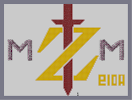Hover over the thumbnail for a full-size version.

Author Slightly_Thwumped_up author:slightly_thwumped_up n-art rated 2007-05-22 3 by 6 people. \$Zelda#Slightly_Thwumped_up#none#00000000000000000000000000000000000000000000000000000000000000000000000000000000000000000000000000000000000000000000000000000000000000000000000000000000000000000000000000000000000000000000000000000000000000000000000000000000000000000000000000000000000000000000000000000000000000000000000000000000000000000000000000000000000000000000000000000000000000000000000000000000000000000000000000000000000000000000000000000000000000000000000000000000000000000000000000000000000000000000000000000000000000000000000000000000000000000000000000000000000000000000000000000000000000000000000000000000000000000000000000000000000000000000000000000000000000000000000000000000000000000000000000000000000000000000000000000000000000000|5^468,564!0^510,192!0^504,192!0^498,192!0^492,192!0^486,192!0^480,192!0^474,192!0^468,192!0^456,192!0^462,192!0^450,192!0^444,192!0^444,186!0^444,180!0^444,174!0^444,168!0^450,168!0^462,168!0^456,168!0^468,168!0^474,168!0^480,168!0^486,168!0^492,168!0^498,168!0^504,168!0^510,168!0^516,168!0^522,168!0^528,168!0^534,168!0^540,168!0^546,168!0^552,168!0^558,168!0^564,168!0^570,168!0^576,168!0^582,168!0^588,168!0^594,168!0^600,168!0^594,174!0^588,180!0^582,186!0^576,192!0^570,198!0^564,204!0^558,210!0^552,216!0^546,222!0^540,228!0^534,234!0^528,240!0^522,246!0^516,252!0^510,258!0^504,264!0^498,270!0^492,276!0^486,282!0^480,288!0^474,294!0^468,300!0^462,306!0^456,312!0^450,318!0^444,324!0^438,330!0^432,336!0^426,342!0^420,348!0^414,354!0^408,360!0^402,366!0^396,372!0^390,378!0^384,384!0^378,390!0^372,396!0^366,402!0^360,408!0^354,414!0^348,420!0^342,426!0^336,432!0^330,438!0^324,444!0^318,450!0^312,456!0^318,456!0^324,456!0^324,456!0^330,456!0^336,456!0^342,456!0^348,456!0^348,456!0^354,456!0^360,456!0^366,456!0^366,456!0^372,456!0^372,462!0^372,468!0^372,474!0^372,474!0^372,480!0^366,480!0^360,480!0^354,480!0^348,480!0^342,480!0^336,480!0^336,480!0^330,480!0^324,480!0^324,480!0^318,480!0^312,480!0^306,480!0^300,480!0^300,480!0^288,480!0^288,480!0^282,480!0^276,480!0^270,480!0^264,480!0^294,480!0^258,480!0^246,480!0^240,480!0^234,480!0^228,480!0^222,480!0^216,480!0^252,480!0^222,474!0^228,468!0^234,462!0^240,456!0^246,450!0^252,444!0^258,438!0^258,438!0^264,432!0^270,426!0^276,420!0^282,414!0^288,408!0^294,402!0^306,390!0^312,384!0^318,378!0^324,372!0^330,366!0^336,360!0^342,354!0^348,348!0^354,342!0^360,336!0^366,330!0^372,324!0^378,318!0^384,312!0^396,306!0^390,306!0^396,300!0^402,294!0^408,288!0^414,282!0^420,276!0^426,270!0^432,264!0^438,258!0^444,252!0^450,246!0^456,240!0^462,234!0^468,228!0^474,222!0^480,216!0^486,210!0^492,204!0^498,198!0^306,390!0^300,396!0^372,192!0^372,186!0^372,180!0^372,174!0^372,168!0^366,168!0^360,168!0^354,168!0^348,168!0^342,168!0^336,168!0^324,168!0^318,174!0^318,168!0^330,168!0^312,168!0^306,168!0^300,168!0^366,192!0^360,192!0^354,198!0^348,204!0^342,210!0^336,216!0^330,222!0^324,228!0^318,234!0^312,240!0^306,246!0^300,252!0^294,258!0^282,270!0^288,264!0^276,276!0^270,282!0^264,288!0^258,294!0^252,300!0^246,306!0^234,300!0^234,294!0^300,174!0^294,180!0^294,186!0^294,186!0^288,192!0^288,198!0^282,204!0^282,210!0^276,216!0^276,222!0^270,222!0^270,228!0^270,228!0^270,234!0^264,240!0^264,246!0^264,252!0^258,252!0^258,252!0^258,258!0^258,264!0^258,270!0^252,264!0^252,270!0^252,276!0^246,276!0^246,282!0^582,336!0^456,456!0^462,450!0^468,444!0^474,438!0^480,432!0^486,426!0^492,420!0^498,414!0^504,408!0^510,402!0^516,396!0^522,390!0^528,384!0^534,378!0^540,372!0^546,366!0^552,360!0^558,354!0^576,336!0^510,480!0^504,480!0^498,480!0^492,480!0^486,480!0^480,480!0^468,480!0^468,480!0^456,480!0^450,480!0^450,480!0^444,480!0^444,474!0^444,474!0^444,462!0^444,462!0^444,456!0^450,456!0^444,468!0^474,480!0^462,480!12^384,288!12^384,282!12^384,276!12^384,276!12^384,270!12^384,264!12^384,264!12^384,258!12^384,258!12^384,252!12^384,246!12^384,246!12^384,240!12^384,234!12^384,234!12^384,228!12^384,222!12^384,210!12^384,204!12^384,198!12^384,192!12^384,180!12^384,174!12^384,168!12^378,156!12^372,144!12^378,150!12^366,144!12^360,144!12^360,144!12^354,144!12^354,144!12^354,144!12^384,162!12^348,144!12^342,144!12^336,144!12^330,138!12^324,132!12^318,126!12^312,120!12^318,120!12^324,120!12^330,120!12^336,120!12^342,120!12^348,120!12^354,120!12^360,120!12^366,120!12^372,120!12^378,120!12^384,120!12^384,114!12^384,108!12^384,102!12^384,96!12^384,90!12^384,84!12^384,78!12^384,72!12^384,72!12^384,66!12^384,60!12^384,54!12^384,48!12^384,42!12^384,36!12^384,30!12^384,30!12^384,24!12^504,120!12^498,120!12^492,120!12^486,120!12^480,120!12^474,120!12^474,120!12^468,120!12^462,120!12^462,120!12^456,120!12^450,120!12^444,120!12^444,120!12^438,120!12^432,120!12^432,114!12^432,102!12^432,102!12^432,96!12^432,90!12^432,78!12^432,72!12^432,66!12^432,60!12^432,54!12^432,54!12^432,48!12^432,42!12^432,36!12^432,30!12^432,24!12^432,84!12^432,108!12^384,216!12^384,186!12^432,234!12^432,240!12^432,228!12^432,222!12^432,216!12^432,210!12^432,204!12^432,198!12^432,192!12^432,186!12^432,186!12^432,180!12^432,174!12^432,168!12^432,162!12^438,156!12^438,150!12^444,144!12^450,144!12^456,144!12^462,144!12^462,144!12^474,144!12^474,144!12^480,144!12^486,138!12^492,132!12^498,126!12^468,144!12^390,282!12^396,276!12^402,270!12^408,264!12^414,258!12^420,252!12^426,246!12^432,366!12^432,372!12^432,384!12^432,390!12^432,396!12^432,402!12^432,414!12^432,426!12^432,438!12^432,450!12^432,462!12^432,468!12^432,474!12^432,486!12^432,498!12^432,510!12^432,522!12^432,528!12^432,516!12^432,504!12^432,492!12^432,480!12^432,456!12^432,444!12^432,432!12^432,420!12^432,408!12^432,378!12^426,366!12^432,360!12^420,372!12^414,378!12^408,390!12^408,384!12^402,390!12^396,396!12^390,402!12^384,414!12^384,408!12^384,420!12^384,426!12^384,432!12^384,438!12^384,444!12^384,444!12^384,450!12^384,456!12^384,456!12^384,462!12^384,468!12^384,474!12^384,480!12^384,486!12^384,498!12^384,504!12^384,510!12^384,516!12^384,492!12^384,522!12^384,528!12^426,534!12^426,540!12^420,546!12^420,552!12^414,558!12^414,564!12^408,570!12^408,576!12^402,564!12^402,558!12^396,552!12^396,546!12^390,540!12^390,534!12^426,528!12^426,522!12^426,516!12^426,516!12^426,510!12^426,504!12^426,498!12^426,492!12^426,486!12^426,480!12^426,480!12^426,474!12^426,468!12^426,468!12^426,462!12^426,456!12^426,450!12^426,444!12^426,438!12^426,432!12^426,426!12^426,426!12^426,420!12^426,408!12^426,402!12^426,396!12^426,390!12^426,384!12^426,378!12^426,378!12^426,372!12^426,408!12^426,408!12^426,414!12^420,540!12^420,534!12^420,528!12^420,522!12^420,516!12^420,510!12^420,504!12^420,498!12^420,492!12^420,492!12^420,486!12^420,480!12^420,474!12^420,468!12^420,462!12^420,456!12^420,450!12^420,438!12^420,432!12^420,426!12^420,420!12^420,414!12^420,408!12^420,408!12^420,402!12^420,396!12^420,390!12^420,384!12^420,378!12^420,444!12^414,552!12^414,540!12^414,534!12^414,528!12^414,522!12^414,510!12^414,510!12^414,504!12^414,492!12^414,486!12^414,480!12^414,474!12^414,462!12^414,450!12^414,438!12^414,432!12^414,426!12^414,414!12^414,408!12^414,402!12^414,390!12^414,384!12^414,396!12^414,420!12^414,444!12^414,456!12^414,468!12^414,498!12^414,516!12^414,546!12^408,564!12^408,558!12^408,552!12^408,546!12^408,534!12^408,528!12^408,522!12^408,522!12^408,516!12^408,510!12^408,504!12^408,498!12^408,498!12^408,492!12^408,480!12^408,480!12^408,468!12^408,456!12^408,450!12^408,438!12^408,432!12^408,426!12^408,414!12^408,408!12^408,396!12^408,420!12^408,402!12^408,444!12^408,462!12^408,474!12^408,486!12^408,540!12^402,552!12^402,540!12^402,534!12^402,534!12^402,528!12^402,516!12^402,510!12^402,510!12^402,504!12^402,492!12^402,486!12^402,474!12^402,462!12^402,456!12^402,456!12^402,444!12^402,438!12^402,432!12^402,426!12^402,414!12^402,408!12^402,402!12^402,396!12^402,420!12^402,450!12^402,468!12^402,480!12^402,498!12^402,522!12^402,546!12^396,540!12^396,528!12^396,522!12^396,516!12^396,510!12^396,504!12^396,492!12^396,492!12^396,480!12^396,474!12^396,474!12^396,462!12^396,456!12^396,450!12^396,444!12^396,438!12^396,432!12^396,420!12^396,414!12^396,408!12^396,402!12^396,426!12^396,468!12^396,486!12^396,498!12^396,534!12^390,528!12^390,516!12^390,510!12^390,504!12^390,492!12^390,486!12^390,480!12^390,468!12^390,456!12^390,444!12^390,432!12^390,426!12^390,414!12^390,408!12^390,420!12^390,438!12^390,450!12^390,462!12^390,474!12^390,498!12^390,522!12^390,276!12^390,276!12^390,264!12^390,264!12^390,258!12^390,258!12^390,252!12^390,246!12^390,240!12^390,240!12^390,228!12^390,222!12^390,216!12^390,210!12^390,204!12^390,198!12^390,192!12^390,186!12^390,180!12^390,174!12^390,168!12^390,162!12^390,150!12^390,150!12^390,138!12^390,132!12^390,126!12^390,120!12^390,114!12^390,102!12^390,102!12^390,90!12^390,84!12^390,72!12^390,66!12^390,60!12^390,54!12^390,48!12^390,42!12^390,30!12^390,24!12^396,24!12^402,24!12^408,24!12^414,24!12^420,24!12^426,24!12^426,30!12^426,36!12^426,48!12^426,48!12^426,54!12^426,60!12^426,72!12^426,78!12^426,90!12^426,96!12^426,102!12^426,108!12^426,114!12^426,114!12^426,126!12^426,126!12^426,132!12^426,138!12^426,144!12^426,150!12^426,156!12^426,156!12^426,162!12^426,174!12^426,180!12^426,186!12^426,186!12^426,192!12^426,204!12^426,210!12^426,216!12^426,222!12^426,228!12^426,234!12^426,240!12^426,198!12^426,168!12^426,120!12^426,84!12^426,72!12^426,66!12^426,42!12^390,36!12^390,78!12^390,90!12^390,96!12^390,108!12^390,144!12^390,156!12^384,228!12^390,234!12^390,270!12^384,156!12^384,150!12^384,144!12^384,144!12^384,138!12^384,132!12^384,126!12^378,126!12^378,132!12^378,138!12^378,144!12^372,138!12^366,138!12^354,138!12^360,138!12^348,138!12^342,138!12^336,138!12^336,132!12^342,132!12^348,132!12^354,132!12^360,132!12^366,132!12^372,132!12^372,126!12^366,126!12^360,126!12^354,126!12^348,126!12^342,126!12^336,126!12^330,126!12^330,126!12^324,126!12^330,132!12^492,126!12^486,126!12^480,126!12^474,126!12^468,126!12^468,126!12^462,126!12^462,126!12^456,126!12^450,126!12^444,126!12^438,126!12^432,126!12^432,132!12^432,138!12^432,144!12^432,150!12^432,156!12^438,144!12^438,138!12^438,132!12^444,132!12^450,132!12^450,126!12^456,132!12^456,132!12^462,132!12^468,132!12^474,132!12^486,132!12^486,132!12^480,138!12^474,138!12^468,138!12^468,138!12^456,138!12^456,138!12^450,138!12^444,138!12^462,138!12^480,132!12^420,246!12^420,240!12^420,228!12^420,228!12^420,222!12^420,216!12^420,210!12^420,198!12^420,198!12^420,186!12^420,180!12^420,174!12^420,168!12^420,162!12^420,150!12^420,150!12^420,144!12^420,138!12^420,132!12^420,120!12^420,114!12^420,108!12^420,102!12^420,96!12^420,90!12^420,84!12^420,78!12^420,72!12^420,60!12^420,54!12^420,48!12^420,42!12^420,36!12^420,30!12^414,252!12^414,252!12^414,240!12^414,234!12^414,228!12^414,222!12^414,216!12^414,210!12^414,210!12^414,204!12^414,192!12^414,186!12^414,180!12^414,168!12^414,162!12^414,156!12^414,150!12^414,144!12^414,132!12^414,126!12^414,120!12^414,114!12^414,114!12^414,102!12^414,96!12^414,90!12^414,78!12^414,66!12^414,66!12^414,60!12^414,48!12^414,42!12^414,36!12^414,36!12^414,30!12^408,258!12^408,246!12^408,240!12^408,234!12^408,228!12^408,222!12^408,216!12^408,204!12^408,198!12^408,192!12^408,186!12^408,174!12^408,168!12^408,162!12^408,156!12^408,150!12^408,144!12^408,138!12^408,126!12^408,126!12^408,120!12^408,108!12^408,108!12^408,102!12^408,96!12^408,84!12^408,84!12^408,78!12^408,72!12^408,60!12^408,54!12^408,48!12^408,42!12^408,36!12^408,36!12^408,30!12^402,264!12^402,252!12^402,246!12^402,234!12^402,228!12^402,216!12^402,198!12^402,168!12^402,150!12^402,126!12^402,102!12^402,78!12^402,60!12^402,36!12^402,30!12^402,48!12^402,66!12^402,126!12^402,150!12^402,192!12^408,222!12^408,132!12^408,90!12^402,162!12^402,186!12^402,180!12^402,114!12^402,84!12^408,156!12^408,102!12^402,144!12^402,108!12^402,138!12^396,126!12^396,84!12^396,174!12^396,96!12^396,162!12^396,168!12^396,138!12^396,204!12^396,138!12^396,222!12^396,192!12^396,180!12^396,228!12^396,246!12^396,252!12^396,264!12^396,270!12^396,240!12^396,204!12^396,186!12^396,156!12^396,138!12^396,114!12^396,96!12^396,78!12^396,72!12^396,60!12^396,54!12^396,48!12^396,42!12^396,30!12^420,234!12^414,246!12^408,252!12^402,258!12^396,258!12^402,240!12^396,234!12^402,222!12^396,216!12^396,210!12^402,210!12^408,210!12^420,204!12^414,198!12^420,192!12^402,204!12^396,198!12^408,180!12^414,174!12^402,174!12^420,156!12^414,138!12^420,126!12^420,126!12^402,156!12^396,150!12^396,144!12^396,132!12^402,132!12^396,120!12^402,120!12^408,114!12^414,108!12^390,108!12^396,108!12^396,102!12^402,96!12^396,90!12^402,90!12^414,84!12^414,72!12^420,60!12^420,66!12^408,66!12^402,72!12^396,66!12^402,54!12^414,54!12^402,42!12^396,36!0^450,186!0^462,186!0^462,186!0^468,186!0^474,186!0^480,186!0^486,186!0^492,186!0^498,186!0^504,186!0^516,186!0^522,186!0^534,186!0^534,186!0^546,192!0^552,192!0^564,192!0^570,192!0^570,186!0^576,186!0^570,186!0^564,186!0^558,186!0^558,186!0^552,186!0^552,186!0^546,186!0^540,186!0^540,186!0^528,186!0^516,186!0^510,186!0^456,186!0^450,180!0^456,180!0^456,180!0^462,180!0^462,180!0^468,180!0^474,180!0^480,180!0^486,180!0^492,180!0^492,180!0^504,180!0^510,180!0^516,180!0^528,180!0^534,186!0^540,186!0^552,180!0^558,180!0^570,180!0^576,180!0^582,180!0^564,180!0^546,174!0^546,180!0^534,180!0^522,180!0^498,180!0^588,174!0^576,174!0^576,174!0^570,174!0^564,174!0^564,174!0^558,174!0^540,180!0^540,174!0^534,174!0^528,174!0^522,174!0^516,174!0^510,174!0^498,174!0^492,174!0^486,174!0^474,174!0^462,174!0^456,174!0^450,174!0^468,174!0^480,174!0^504,174!0^552,174!0^588,174!0^366,174!0^360,174!0^354,174!0^348,174!0^342,174!0^336,174!0^330,174!0^324,174!0^312,174!0^306,174!0^300,180!0^306,180!0^312,180!0^312,180!0^324,180!0^318,180!0^330,180!0^330,180!0^336,180!0^342,180!0^348,180!0^354,180!0^354,180!0^360,180!0^366,180!0^366,186!0^360,186!0^354,186!0^354,186!0^348,186!0^342,186!0^342,186!0^336,186!0^336,186!0^330,186!0^324,186!0^318,186!0^312,186!0^306,186!0^300,186!0^294,192!0^300,192!0^306,192!0^312,192!0^318,192!0^324,192!0^330,192!0^336,192!0^342,192!0^348,192!0^354,192!0^516,192!0^522,192!0^528,192!0^534,192!0^546,192!0^540,192!0^558,192!0^582,174!0^330,198!0^318,204!0^306,210!0^300,222!0^282,246!0^276,264!0^270,276!0^258,288!0^234,300!0^294,204!0^288,216!0^288,228!0^282,240!0^276,252!0^270,258!0^288,252!0^294,240!0^312,228!0^324,216!0^336,204!0^312,216!0^294,216!0^342,198!0^336,198!0^318,216!0^306,222!0^300,234!0^288,240!0^282,258!0^270,270!0^264,264!0^288,258!0^276,258!0^264,258!0^282,252!0^276,270!0^270,264!0^282,264!0^264,270!0^264,276!0^264,282!0^258,282!0^258,276!0^252,282!0^252,288!0^252,294!0^246,300!0^240,300!0^240,306!0^234,306!0^348,198!0^342,204!0^336,210!0^330,216!0^324,222!0^318,228!0^312,234!0^306,240!0^300,246!0^294,252!0^270,240!0^276,228!0^264,234!0^258,246!0^252,258!0^246,270!0^240,282!0^330,210!0^330,204!0^324,204!0^324,198!0^318,198!0^312,198!0^312,198!0^306,198!0^300,198!0^294,198!0^300,204!0^306,198!0^306,198!0^306,204!0^312,204!0^318,204!0^324,204!0^324,210!0^318,210!0^312,210!0^318,222!0^312,222!0^306,216!0^300,216!0^300,210!0^294,210!0^288,210!0^288,198!0^288,198!0^288,204!0^276,210!0^282,198!0^288,186!0^294,174!0^306,234!0^306,228!0^300,228!0^294,228!0^294,222!0^288,222!0^282,222!0^282,216!0^282,216!0^300,240!0^294,234!0^288,234!0^288,234!0^276,234!0^282,234!0^276,240!0^276,246!0^270,246!0^270,252!0^294,246!0^288,246!0^282,228!0^420,306!0^456,276!0^474,264!0^498,240!0^534,204!0^534,198!0^504,228!0^474,264!0^426,318!0^366,396!0^324,432!0^336,402!0^354,378!0^396,348!0^426,306!0^384,330!0^348,366!0^330,390!0^348,378!0^360,366!0^390,342!0^384,348!0^342,384!0^360,378!0^426,324!0^366,372!0^354,390!0^444,318!0^384,348!0^414,312!0^402,336!0^444,282!0^426,312!0^438,300!0^504,210!0^432,282!0^492,216!0^432,276!0^504,204!0^450,282!0^504,222!0^444,276!0^468,246!0^462,264!0^528,204!0^486,270!0^528,222!0^444,300!0^450,282!0^372,360!0^288,450!0^342,384!0^276,462!0^312,426!0^270,444!0^258,450!0^336,396!0^276,444!0^312,432!0^324,426!0^282,444!0^294,408!0^360,354!0^294,408!0^324,396!0^324,390!0^300,420!0^390,336!0^366,468!0^354,468!0^354,468!0^348,462!0^336,462!0^330,462!0^318,468!0^312,468!0^288,468!0^276,468!0^276,468!0^258,474!0^246,474!0^234,474!0^228,474!0^366,474!0^360,474!0^360,474!0^354,474!0^348,474!0^342,474!0^342,474!0^336,474!0^330,474!0^324,474!0^324,474!0^318,474!0^312,474!0^300,474!0^294,474!0^288,474!0^288,474!0^282,474!0^276,474!0^270,474!0^264,474!0^252,474!0^240,474!0^306,474!0^360,468!0^348,468!0^342,468!0^336,468!0^330,468!0^324,468!0^306,468!0^300,468!0^294,468!0^282,468!0^270,468!0^264,468!0^258,468!0^252,468!0^246,468!0^240,468!0^234,468!0^366,462!0^360,462!0^354,462!0^342,462!0^324,462!0^318,462!0^312,462!0^306,462!0^300,462!0^294,462!0^288,462!0^282,462!0^270,462!0^264,462!0^258,462!0^252,462!0^246,462!0^240,462!0^312,456!0^306,456!0^306,456!0^300,456!0^294,456!0^288,456!0^282,456!0^276,456!0^270,456!0^270,456!0^264,456!0^258,456!0^258,456!0^252,456!0^246,456!0^312,450!0^306,450!0^306,450!0^300,450!0^294,450!0^282,450!0^276,450!0^270,450!0^264,450!0^252,450!0^258,444!0^264,444!0^288,444!0^294,444!0^300,444!0^300,444!0^312,444!0^312,444!0^318,444!0^318,444!0^306,444!0^564,198!0^558,198!0^558,198!0^552,198!0^546,198!0^540,198!0^528,198!0^516,198!0^504,198!0^522,198!0^510,198!0^558,204!0^552,204!0^546,204!0^540,204!0^522,204!0^516,204!0^510,204!0^498,204!0^552,210!0^540,210!0^546,210!0^534,210!0^528,210!0^522,210!0^516,210!0^510,210!0^498,210!0^492,210!0^546,216!0^540,216!0^534,216!0^522,216!0^516,216!0^504,216!0^504,216!0^498,216!0^498,222!0^498,228!0^498,234!0^504,234!0^504,240!0^504,246!0^504,246!0^504,258!0^498,258!0^498,264!0^498,252!0^498,246!0^504,252!0^510,252!0^510,246!0^510,240!0^510,234!0^510,228!0^510,222!0^516,222!0^516,228!0^516,234!0^516,240!0^522,240!0^522,234!0^522,228!0^522,222!0^528,234!0^528,228!0^516,246!0^510,216!0^528,216!0^540,222!0^534,222!0^534,222!0^534,228!0^564,348!0^570,342!0^522,468!0^522,462!0^528,456!0^528,450!0^534,444!0^534,438!0^540,432!0^540,426!0^546,414!0^546,420!0^552,408!0^552,402!0^558,396!0^558,390!0^564,384!0^564,378!0^570,372!0^570,366!0^576,360!0^576,354!0^582,348!0^582,342!0^588,336!0^516,474!0^516,480!0^498,456!0^510,438!0^528,414!0^552,396!0^558,372!0^540,390!0^522,414!0^498,450!0^486,456!0^486,450!0^522,420!0^534,402!0^498,456!0^474,468!0^462,468!0^462,468!0^480,468!0^510,474!0^498,474!0^498,474!0^492,474!0^486,474!0^480,474!0^474,474!0^468,474!0^462,474!0^456,474!0^450,474!0^504,474!0^516,468!0^504,468!0^504,468!0^498,468!0^492,468!0^486,468!0^468,468!0^456,468!0^450,468!0^510,468!0^516,462!0^510,462!0^510,462!0^504,462!0^498,462!0^492,462!0^492,462!0^486,462!0^480,462!0^480,462!0^474,462!0^474,462!0^474,462!0^468,462!0^462,462!0^456,462!0^450,462!0^522,456!0^516,456!0^510,456!0^504,456!0^492,456!0^480,456!0^474,456!0^468,456!0^462,456!0^522,450!0^516,450!0^510,450!0^504,450!0^492,450!0^480,450!0^474,450!0^468,450!0^528,444!0^522,444!0^522,438!0^516,438!0^516,444!0^516,444!0^516,444!0^510,444!0^510,444!0^504,444!0^498,444!0^498,444!0^492,444!0^486,444!0^480,444!0^480,444!0^474,444!0^528,438!0^504,438!0^498,438!0^492,438!0^486,438!0^480,438!0^534,432!0^534,426!0^534,426!0^534,420!0^534,414!0^534,408!0^528,408!0^522,408!0^516,408!0^516,414!0^516,414!0^516,420!0^516,426!0^516,426!0^516,432!0^510,432!0^504,432!0^498,432!0^492,432!0^492,426!0^498,426!0^498,426!0^504,420!0^504,420!0^510,426!0^504,426!0^486,432!0^498,420!0^504,414!0^510,408!0^510,414!0^510,420!0^528,420!0^528,426!0^528,432!0^522,432!0^522,426!0^540,420!0^540,414!0^540,408!0^540,402!0^540,396!0^534,396!0^528,396!0^528,402!0^522,402!0^516,402!0^522,396!0^546,408!0^546,402!0^546,396!0^546,390!0^546,384!0^540,384!0^534,384!0^534,390!0^528,390!0^552,390!0^552,384!0^552,378!0^546,378!0^540,378!0^558,384!0^558,378!0^546,372!0^552,372!0^564,372!0^564,366!0^564,366!0^558,366!0^552,366!0^570,360!0^564,360!0^558,360!0^564,354!0^570,354!0^570,348!0^576,348!0^576,342!0^492,222!0^492,228!0^492,234!0^492,240!0^492,246!0^492,252!0^492,258!0^492,264!0^492,270!0^486,264!0^486,258!0^486,252!0^486,246!0^486,240!0^486,234!0^486,228!0^486,222!0^486,216!0^486,276!0^480,282!0^480,276!0^480,270!0^480,258!0^480,246!0^480,240!0^480,234!0^480,228!0^480,222!0^480,252!0^480,264!0^474,270!0^474,276!0^474,282!0^474,288!0^474,258!0^474,252!0^474,246!0^474,240!0^474,234!0^474,228!0^438,306!0^432,306!0^432,294!0^426,294!0^414,300!0^408,306!0^402,318!0^414,324!0^414,330!0^414,342!0^414,348!0^414,336!0^402,348!0^396,354!0^390,366!0^384,366!0^384,372!0^372,378!0^366,378!0^366,384!0^360,384!0^354,390!0^348,396!0^348,402!0^348,402!0^342,408!0^330,414!0^330,414!0^324,414!0^312,414!0^306,420!0^300,426!0^300,432!0^288,432!0^282,432!0^264,438!0^270,438!0^276,438!0^288,438!0^282,438!0^294,438!0^300,438!0^306,438!0^312,438!0^312,438!0^318,438!0^324,438!0^318,426!0^318,426!0^318,420!0^312,420!0^306,426!0^306,432!0^318,432!0^270,432!0^276,432!0^276,426!0^282,426!0^282,420!0^288,420!0^288,414!0^294,414!0^300,414!0^306,414!0^306,408!0^312,408!0^318,408!0^324,408!0^324,414!0^324,420!0^330,420!0^330,426!0^336,426!0^336,420!0^342,420!0^342,414!0^348,414!0^348,408!0^354,408!0^354,402!0^360,402!0^360,396!0^366,396!0^366,390!0^360,390!0^360,396!0^354,396!0^330,432!0^318,414!0^294,420!0^294,426!0^294,432!0^288,426!0^336,414!0^336,408!0^330,408!0^330,402!0^324,402!0^318,402!0^312,402!0^306,402!0^300,402!0^300,408!0^342,402!0^342,396!0^342,390!0^336,390!0^336,390!0^336,384!0^330,384!0^324,384!0^318,384!0^318,390!0^318,396!0^312,396!0^306,396!0^312,390!0^330,396!0^348,390!0^348,384!0^354,384!0^372,390!0^372,378!0^372,384!0^378,384!0^378,378!0^378,372!0^378,366!0^378,360!0^378,354!0^378,354!0^378,348!0^378,342!0^378,336!0^378,330!0^378,324!0^372,372!0^372,366!0^366,366!0^366,354!0^366,354!0^366,348!0^366,336!0^366,336!0^372,330!0^372,336!0^372,336!0^372,342!0^372,348!0^372,348!0^354,366!0^354,360!0^354,354!0^354,348!0^360,372!0^354,372!0^354,366!0^348,366!0^348,360!0^348,366!0^348,366!0^348,372!0^348,378!0^342,378!0^342,378!0^336,378!0^336,378!0^330,378!0^330,372!0^336,372!0^342,372!0^342,366!0^342,366!0^342,360!0^336,366!0^324,378!0^348,354!0^360,348!0^360,342!0^372,342!0^366,342!0^366,360!0^360,360!0^384,378!0^390,372!0^396,366!0^396,360!0^390,360!0^390,354!0^390,348!0^384,342!0^384,336!0^384,354!0^384,360!0^372,354!0^474,282!0^468,288!0^456,294!0^450,306!0^444,306!0^432,324!0^432,324!0^420,336!0^420,336!0^420,330!0^420,324!0^420,318!0^414,318!0^408,318!0^408,324!0^408,330!0^408,336!0^408,342!0^408,348!0^408,354!0^402,354!0^402,360!0^402,342!0^396,342!0^396,336!0^396,324!0^396,318!0^396,312!0^390,312!0^390,318!0^384,318!0^384,324!0^390,324!0^390,330!0^396,330!0^402,330!0^402,324!0^420,342!0^426,336!0^426,330!0^432,330!0^438,324!0^438,318!0^438,312!0^432,312!0^432,318!0^444,312!0^450,312!0^456,306!0^456,300!0^450,300!0^450,294!0^444,294!0^438,294!0^438,288!0^438,282!0^438,276!0^438,270!0^438,264!0^444,264!0^450,264!0^456,264!0^456,258!0^456,252!0^456,246!0^462,246!0^462,252!0^462,258!0^468,258!0^468,264!0^468,270!0^468,276!0^468,282!0^462,282!0^456,282!0^456,288!0^450,288!0^444,288!0^468,294!0^462,294!0^462,300!0^462,288!0^462,276!0^462,270!0^456,270!0^450,270!0^444,276!0^444,270!0^450,276!0^468,252!0^468,252!0^468,234!0^468,240!0^462,240!0^450,252!0^450,258!0^444,258!0^432,270!0^426,276!0^426,282!0^426,288!0^432,288!0^432,300!0^426,300!0^420,300!0^420,294!0^420,288!0^414,288!0^414,294!0^408,294!0^408,300!0^402,300!0^402,306!0^402,312!0^408,312!0^414,306!0^420,312!0^420,282!0^240,288!0^240,294!0^246,294!0^246,288!0^240,312!0^234,312!0^228,312!0^228,306!12^384,294!12^390,288!12^396,282!12^402,276!12^408,270!12^414,264!12^420,258!12^426,252!12^432,246!12^432,354!12^426,360!12^420,366!12^414,372!12^408,378!12^402,384!12^396,390!12^390,396!12^384,402!0^600,480!0^624,462!0^624,456!0^618,456!0^612,456!0^606,456!0^600,456!0^600,462!0^600,468!0^600,486!0^600,492!0^600,498!0^624,480!0^624,468!0^624,474!0^618,480!0^612,480!0^606,480!0^600,474!0^600,504!0^606,504!0^612,504!0^612,504!0^618,504!0^624,504!0^624,510!0^618,510!0^612,510!0^612,510!0^606,510!0^600,510!0^594,510!0^594,504!0^594,498!0^594,492!0^594,480!0^594,486!0^594,474!0^594,468!0^594,462!0^594,456!0^594,450!0^600,450!0^606,450!0^612,450!0^618,450!0^624,450!0^630,450!0^630,456!0^630,462!0^630,468!0^630,474!0^630,480!0^630,486!0^624,486!0^612,486!0^606,486!0^618,486!0^630,504!0^630,510!0^648,456!0^648,462!0^648,462!0^648,468!0^648,474!0^648,480!0^648,486!0^648,492!0^648,498!0^648,504!0^648,510!0^654,510!0^654,510!0^654,504!0^654,498!0^654,486!0^654,480!0^654,474!0^654,468!0^654,462!0^654,456!0^654,450!0^648,450!0^654,492!0^672,450!0^672,456!0^672,462!0^672,468!0^672,468!0^672,474!0^672,480!0^672,486!0^672,492!0^672,498!0^672,504!0^672,510!0^678,510!0^678,504!0^678,498!0^678,492!0^678,486!0^678,480!0^678,474!0^678,468!0^678,462!0^678,456!0^678,450!0^684,450!0^690,450!0^696,450!0^696,456!0^696,462!0^696,468!0^696,474!0^696,480!0^696,486!0^696,492!0^696,498!0^690,510!0^684,510!0^684,504!0^690,504!0^690,456!0^684,456!0^696,510!0^696,504!0^714,510!0^714,504!0^714,498!0^714,492!0^714,486!0^714,480!0^714,474!0^714,468!0^714,462!0^714,450!0^714,456!0^720,450!0^720,456!0^720,462!0^720,468!0^720,474!0^720,480!0^720,486!0^720,492!0^720,498!0^720,504!0^720,510!0^726,450!0^732,450!0^738,450!0^744,450!0^744,456!0^744,462!0^744,468!0^744,474!0^744,480!0^744,486!0^744,492!0^744,498!0^744,504!0^744,510!0^738,510!0^738,504!0^738,498!0^738,492!0^738,486!0^738,480!0^738,474!0^738,468!0^738,468!0^738,462!0^738,456!0^732,492!0^732,492!0^726,492!0^726,498!0^732,498!10^187,246!10^187,253!10^187,261!10^187,271!10^187,279!10^187,288!10^187,299!10^188,308!10^188,317!10^188,327!10^188,339!10^188,351!10^188,358!10^180,359!10^173,359!10^179,349!10^179,338!10^69,269!10^69,277!10^69,284!10^69,293!10^69,301!10^69,312!10^69,320!10^69,328!10^69,337!10^69,347!10^69,356!10^120,310!10^126,302!10^132,298!10^139,291!10^144,283!10^150,277!10^155,272!10^163,266!10^116,287!10^111,282!10^105,276!10^99,270!10^91,261!10^84,256!10^78,249!10^73,243!10^68,307!3^179,328!3^179,317!3^179,309!3^179,300!3^179,287!3^179,276!3^179,266!3^179,252!3^179,243!3^179,260!3^171,350!3^171,341!3^171,332!3^171,324!3^171,317!3^171,310!3^171,305!3^171,298!3^171,292!3^171,284!3^171,277!3^171,268!3^171,260!3^171,252!3^171,243!3^163,255!3^156,260!3^152,264!3^149,268!3^144,273!3^138,281!3^133,289!3^125,298!3^122,300!3^117,303!3^124,281!3^131,274!3^137,265!3^150,255!3^161,246!3^157,250!3^114,296!3^109,291!3^105,286!3^102,281!3^96,277!3^91,273!3^87,268!3^80,260!3^75,255!3^69,250!3^62,242!3^51,242!3^108,302!3^101,292!3^91,285!3^83,276!3^75,265!3^71,259!3^62,251!3^62,256!3^62,260!3^62,263!3^62,266!3^62,272!3^62,279!3^62,285!3^62,292!3^62,301!3^62,310!3^62,318!3^62,326!3^62,335!3^62,343!3^62,348!3^62,349!3^62,355!3^53,357!3^53,347!3^53,336!3^53,327!3^53,316!3^53,307!3^53,297!3^53,287!3^53,274!3^53,260!3^53,249!10^738,354!10^739,342!10^739,334!10^739,318!10^739,325!10^739,308!10^739,298!10^739,287!10^739,275!10^739,266!10^739,255!10^739,245!10^673,305!10^679,299!10^686,292!10^691,285!10^699,282!10^705,275!10^713,269!10^718,263!10^671,288!10^665,282!10^658,275!10^651,268!10^644,263!10^638,254!10^630,247!10^624,241!10^624,269!10^624,277!10^624,286!10^624,293!10^624,301!10^624,307!10^624,318!10^624,323!10^624,331!10^624,340!10^624,350!10^624,357!10^624,313!3^614,359!3^614,355!3^614,349!3^614,346!3^614,340!3^614,335!3^614,331!3^614,328!3^614,323!3^614,316!3^614,309!3^614,302!3^614,291!3^614,281!3^614,273!3^614,265!3^614,276!3^614,289!3^614,259!3^614,253!3^614,247!3^615,245!3^622,247!3^624,251!3^626,254!3^632,260!3^635,264!3^639,267!3^642,267!3^651,274!3^653,280!3^656,283!3^661,286!3^666,291!3^674,297!3^669,299!3^674,291!3^679,287!3^684,284!3^690,278!3^694,274!3^701,267!3^705,264!3^711,262!3^716,260!3^732,244!3^733,253!3^733,260!3^733,270!3^733,270!3^733,281!3^733,290!3^733,303!3^733,311!3^733,319!3^733,330!3^733,338!3^733,341!3^733,352!3^733,355!3^722,355!3^722,345!3^722,335!3^722,325!3^722,313!3^722,303!3^722,291!3^722,279!3^722,270!3^722,258!3^722,246!3^715,249!3^705,254!3^693,261!3^681,272!3^675,277!3^662,302!3^655,293!3^647,287!3^640,280!3^632,271!3^625,265!3^604,245!3^604,256!3^604,265!3^604,270!3^604,279!3^604,285!3^604,296!3^604,307!3^604,316!3^604,326!3^604,339!3^603,352# DED to legend of Zelda Majora's mask (not numa user).

## Other maps by this author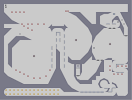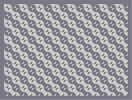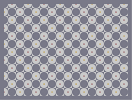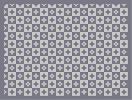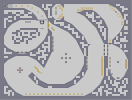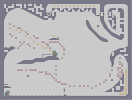gersand fernad tileset 1 tileset 2 tileset 3 topsie go go go